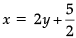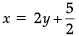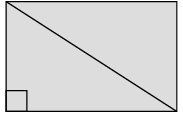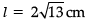Test: Quadrilaterals- Case Based Type Questions- 1

# Test: Quadrilaterals- Case Based Type Questions- 1 - Class 9

Test Description

## 10 Questions MCQ Test Additional Practice for Class 9 - Test: Quadrilaterals- Case Based Type Questions- 1

Test: Quadrilaterals- Case Based Type Questions- 1 for Class 9 2023 is part of Additional Practice for Class 9 preparation. The Test: Quadrilaterals- Case Based Type Questions- 1 questions and answers have been prepared according to the Class 9 exam syllabus.The Test: Quadrilaterals- Case Based Type Questions- 1 MCQs are made for Class 9 2023 Exam. Find important definitions, questions, notes, meanings, examples, exercises, MCQs and online tests for Test: Quadrilaterals- Case Based Type Questions- 1 below.
 1 Crore+ students have signed up on EduRev. Have you?
Test: Quadrilaterals- Case Based Type Questions- 1 - Question 1

### Harish makes a poster in the shape of a parallelogram on the topic SAVE ELECTRICITY for an inter school competition as shown in the follow figure.Q. If AB = (2y – 3) and CD = 5 cm then what is the value of y?

Detailed Solution for Test: Quadrilaterals- Case Based Type Questions- 1 - Question 1
AB = CD

⇒ 2y – 3 = 5

⇒ 2y = 8

⇒ y = 4

Test: Quadrilaterals- Case Based Type Questions- 1 - Question 2

### Harish makes a poster in the shape of a parallelogram on the topic SAVE ELECTRICITY for an inter school competition as shown in the follow figure.Q. If ∠B = (2y)° and ∠D = (3y – 6)°, then find the value of y.

Detailed Solution for Test: Quadrilaterals- Case Based Type Questions- 1 - Question 2
∠B = ∠D

(opposite angles of a parallelogram are equal)

⇒ 2y = 3y – 6

⇒ 2y – 3y = – 6

⇒ – y = – 6

⇒ y = 6

Test: Quadrilaterals- Case Based Type Questions- 1 - Question 3

### Harish makes a poster in the shape of a parallelogram on the topic SAVE ELECTRICITY for an inter school competition as shown in the follow figure.Q. If ∠ A = (4x + 3)° and ∠D = (5x – 3)°, then find the measure of ∠B

Detailed Solution for Test: Quadrilaterals- Case Based Type Questions- 1 - Question 3
Since, ABCD is a parallelogram.

∠A + ∠D = 180°

(4x + 3)° + (5x – 3)° = 180°

9x = 180°

x = 20

∠D = (5x – 3)° = 97°

∠D = ∠B

(opposite angles of a parallelogram are equal)

Thus, ∠B = 97°

Test: Quadrilaterals- Case Based Type Questions- 1 - Question 4

Harish makes a poster in the shape of a parallelogram on the topic SAVE ELECTRICITY for an inter school competition as shown in the follow figure.Q. If ∠A = (2x – 3)° and ∠C = (4y + 2)°, then find how x and y relate.

Detailed Solution for Test: Quadrilaterals- Case Based Type Questions- 1 - Question 4∠A = ∠C

(opposite angles of a parallelogram are equal)

⇒ 2x – 3 = 4y + 2

⇒ 2x = 4y + 5Test: Quadrilaterals- Case Based Type Questions- 1 - Question 5

Harish makes a poster in the shape of a parallelogram on the topic SAVE ELECTRICITY for an inter school competition as shown in the follow figure.Q. Which mathematical concept is used here?

Detailed Solution for Test: Quadrilaterals- Case Based Type Questions- 1 - Question 5
Here, we used Properties of a parallelogram Concept.

The properties of a parallelogram are as follows:

• The opposite sides of a parallelogram are equal.

• The opposite angles of a parallelogram are equal.

• The consecutive angle of a parallelogram is supplementary. If one angle is a right angle, all the angles are right angles in a parallelogram.

• The diagonals of a parallelogram bisect each other.

• Each diagonal of a parallelogram bisects it into two congruent triangles.

• If one pair of opposite sides of a quadrilateral is equal and parallel, then the quadrilateral is a parallelogram.

Test: Quadrilaterals- Case Based Type Questions- 1 - Question 6

During maths lab activity, teacher gives four sticks of lengths 6 cm, 6 cm, 4 cm and 4 cm to each student to make different types of quadrilateral.

She asks following questions from the students:

Q. A student formed a rectangle with these sticks. What is the length of the diagonal of the rectangle formed by the student?

Detailed Solution for Test: Quadrilaterals- Case Based Type Questions- 1 - Question 6
All the angles of a rectangle are right angle. So, diagonal of a rectangle divides it into two right angled triangles. Thus,62 + 42 = l2

36 + 16 = l2

l2 = 52Test: Quadrilaterals- Case Based Type Questions- 1 - Question 7

During maths lab activity, teacher gives four sticks of lengths 6 cm, 6 cm, 4 cm and 4 cm to each student to make different types of quadrilateral.

She asks following questions from the students:

Q. Write the name of quadrilateral that can be formed with these sticks.

Detailed Solution for Test: Quadrilaterals- Case Based Type Questions- 1 - Question 7
Opposite sides of a parallelogram and a rectangle are equal. Also, adjacent sides of kite are equal. Thus, kite, rectangle and parallelogram can be formed with the sticks of lengths 6 cm, 6 cm, 4 cm and 4 cm. Hence, option (c) is correct.
Test: Quadrilaterals- Case Based Type Questions- 1 - Question 8

During maths lab activity, teacher gives four sticks of lengths 6 cm, 6 cm, 4 cm and 4 cm to each student to make different types of quadrilateral.

She asks following questions from the students:

Q. How many types of quadrilaterals can be possible?

Detailed Solution for Test: Quadrilaterals- Case Based Type Questions- 1 - Question 8
3 types of quadrilaterals can be possible here.
Test: Quadrilaterals- Case Based Type Questions- 1 - Question 9

During maths lab activity, teacher gives four sticks of lengths 6 cm, 6 cm, 4 cm and 4 cm to each student to make different types of quadrilateral.

She asks following questions from the students:

Q. Which statement is incorrect ?

Detailed Solution for Test: Quadrilaterals- Case Based Type Questions- 1 - Question 9
A trapezium has only one pair of parallel sides. Thus, it cannot be a parallelogram.
Test: Quadrilaterals- Case Based Type Questions- 1 - Question 10

During maths lab activity, teacher gives four sticks of lengths 6 cm, 6 cm, 4 cm and 4 cm to each student to make different types of quadrilateral.

She asks following questions from the students:

Q. A diagonal of a parallelogram divides it into two _______ triangles.

Detailed Solution for Test: Quadrilaterals- Case Based Type Questions- 1 - Question 10
Two triangles are said to be congruent if they are of the same size and same shape. Two congruent triangles have the same area and perimeter. All the sides and angles of a congruent triangle are equal to the corresponding sides and angles of its congruent triangle. We can prove the congruency of any two triangles by using five different properties, which are - SSS, SAS, AAS, ASA, and RHS.

## Additional Practice for Class 9

266 docs|65 tests